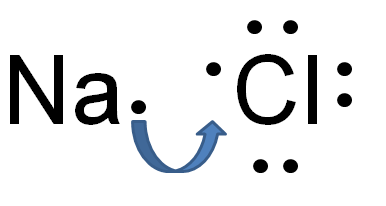Ionic Bonding
4 years ago
sandifaulkner
Save
Edit
Host a game
Live GameLive
Homework
Solo Practice
Practice32 QuestionsShow answers
• Question 1
10 seconds
Q. Ionic bonds form when ions share electrons.
True
False
• Question 2
10 seconds
Q. In sodium chloride, sodium gives an electron to chlorine.
True
False
• Question 3
20 seconds
Q. Ionic bonds form only between atoms of nonmetals.
True
False
• Question 4
20 seconds
Q. The amount of energy needed to form an ion depends only on the number of valence electrons.
True
False
• Question 5
30 seconds
Q. Francium has the same number of valence electrons as lithium.
True
False
• Question 6
20 seconds
Q. Alkali metals release the most energy when they become ions.
True
False
• Question 7
30 seconds
Q. When an atom of iodine becomes an ion, it is named iodide.
True
False
• Question 8
30 seconds
Q. Salt consists of molecules of sodium and chloride atoms.
True
False
• Question 9
30 seconds
Q. Ionic compounds are usually liquids at room temperature.
True
False
• Question 10
20 seconds
Q. Water is an example of an ionic compound.
True
False
• Question 11
30 seconds
Q. When metallic elements become ions they:
gain electrons
become positively charged
become negatively charged
have no charge
• Question 12
30 seconds
Q. Which two elements could form an ionic compound?
#6 carbon and #8 oxygen
#1 hydrogen and #7 nitrogen
#3 lithium and #9 fluorine
#5 boron and #10 neon
• Question 13
30 seconds
Q. Which two elements could form an ionic compound?
#6 carbon and #8 oxygen
#1 hydrogen and #7 nitrogen
#3 lithium and #9 fluorine
#5 boron and #10 neon
• Question 14
30 seconds
Q. Which of the following compounds is not an ionic compound?
barium oxide
lithium oxide
carbon dixoide
calcium chloride
• Question 15
30 seconds
Q. Which statement about energy and ionic bonds is true?
It takes energy to form a negative ion.
Halogens need the most energy to become ions.
It takes energy to remove valence electrons from an atom.
It takes more energy to gain two electrons than one.
• Question 16
30 seconds
Q. Properties of ionic compounds include:
high melting points
high boiling points
brittleness
all of the above
• Question 17
30 seconds
Q. Ionic compounds are good conductors of electricity when they are:
shaped into wires.
dissolved in water.
formed into crystals.
made of two metals.
• Question 18
30 seconds
Q. A dissolved ionic compound is a(an):
ion
crystal
electrolyte
ionic bond
• Question 19
30 seconds
Q. In which of the following elements is the valence electron farthest from the nucleus?
#3 lithium
#11 sodium
#19 potassium
#37 rubidium
• Question 20
30 seconds
Q. A unique substance that forms when a metal and a nonmetal combine chemically is a(an):
ion
ionic bond
crystal
ionic compounds
• Question 21
30 seconds
Q. Which of the following is an example of an alkaline earth metal?
#19 potassium
#3 lithium
#56 barium
#57 lanthanum
• Question 22
30 seconds
Q. The force of attraction that holds together positive and negative ions is a(an):
ionic compound
ionic bond
electrolyte
crystal
• Question 23
30 seconds
Q. Which of the following elements would form a negative ion?
#13 aluminum
#32 germanium
#53 iodine
#26 iron
• Question 24
30 seconds
Q. The charged particle that is formed when an atom gains or loses valence electrons is a(an):
ion
catalyst
electrolyte
molecule
• Question 25
30 seconds
Q. How many electrons does #15 phosphorus need to gain to be stable?
one
two
three
four
• Question 26
30 seconds
Q. The structure that forms when positive and negative ions bond together is a(an):
ionic compound
crystal
electrolyte
ion
• Question 27
30 seconds
Q. Energy is released when an atom ______ an electron and becomes an ion.
gives
gains
• Question 28
30 seconds
Q. When an ionic compound is named, the ______ is always named first.
positive ion
negative ion
• Question 29
30 seconds
Q. In the ionic compound iron bromide, the negative ion is
iron
bromide
• Question 30
30 seconds
Q. When writing the name of an ionic compound, the ________ is always written first.
metal
nonmetal
• Question 31
30 seconds
Q. When writing the name of an ionic compound, the negative ion (nonmetal) is usually written with which suffix?
-ate
-ionate
-ide
-apt
• Question 32
30 seconds
Q. What type of elements are involved in a covalent bond?